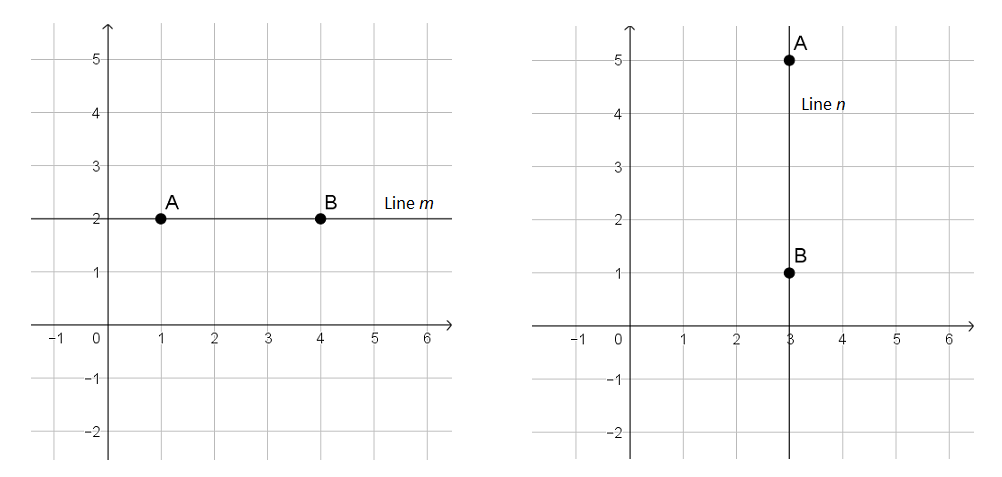# Linear Relationships

## Objective

Determine slope from coordinate points. Find slope of horizontal and vertical lines.

## Common Core Standards

### Core Standards

?

• 8.EE.B.6 — Use similar triangles to explain why the slope m is the same between any two distinct points on a non-vertical line in the coordinate plane; derive the equation y = mx for a line through the origin and the equation y = mx + b for a line intercepting the vertical axis at b.

• 8.F.B.4 — Construct a function to model a linear relationship between two quantities. Determine the rate of change and initial value of the function from a description of a relationship or from two (x, y) values, including reading these from a table or from a graph. Interpret the rate of change and initial value of a linear function in terms of the situation it models, and in terms of its graph or a table of values.

?

• 7.NS.A.1

• 7.NS.A.2.B

## Criteria for Success

?

1. Find the slope of a line using values represented in a table.
2. Find the slope of a line that passes through two given coordinate points.
3. Understand the slope of a horizontal line is 0 and the slope of a vertical line is undefined.

## Tips for Teachers

?

In determining slope from coordinate points, students will need a strong grasp of adding and subtracting signed numbers (standard 7.NS.1). If needed, review these concepts and skills prior to this lesson.

#### Fishtank Plus

• Problem Set
• Student Handout Editor
• Vocabulary Package

## Anchor Problems

?

### Problem 1

The table below shows some solution values for the equation ${{y=-3x+2}}$.

 $x$ $y$ -2 8 -1 5 0 2 1 -1

Use the values in the table to determine the slope of the line represented by ${{y=-3x+2}}$.

### Problem 2

Find the slope of the line between the points (-1, 3) and (5, 11).

Is the point (-4, -1) on the same line as the other two points? Use slope to justify your answer.

### Problem 3

Find the slope of line $m$ and line $n$, shown below.## Problem Set

?The following resources include problems and activities aligned to the objective of the lesson that can be used to create your own problem set.

• Challenge: Line $k$ has a slope of $-\frac{1}{2}$. It passes through the points $(x, -2)$ and $(-3, \frac{1}{4})$. Find the value of $x$ in the coordinate point.

?

Samantha found the slope of the line that passed through the points ${(2, 6)}$ and ${(-4, 8)}$. Her work is shown below.

${{8-6\over {2-(-4)}} = {2\over 2+4 }= {2\over 6} = {1\over3}}$

Samantha made an error in her work. Describe the error and then find the correct slope of the line through the two given points.

?